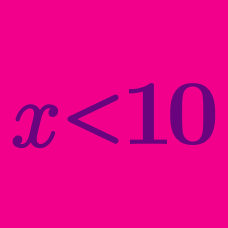Algebra

# Linear Inequalities Word Problems

Consider two successive even numbers. If 10 is subtracted from 4 times the smaller one, then the resulting value is smaller than 2 times the larger one. What is the maximum possible value of the sum of the two successive even numbers?

Adam took an exam with $$20$$ questions, and answered all of the $$20$$ questions. He gets $$5$$ points for a right answer and $$2$$ points taken off for a wrong answer. If Adam's score in the exam is between $$45$$ and $$80$$ exclusive, what is the difference between the maximum possible number of right answers $$(M)$$ and minimum possible number of right answers $$(m)?$$

A pair of jeans at Kim's Clothing which was priced $$30$$% above the merchant's cost is now on sale for $$20$$% off. At the sale price, the profit margin per unit is at least $$$7$$. If the merchant's cost of a pair of jeans is at least$$$x$$, what is $$x$$?

Let $$200n(400-n)$$ represent the total revenue (in dollars) of a company from selling $$n$$ units of the machine they produce, where $$0\le n \le 200.$$ If it costs $$30000$$ dollars to produce one unit of the machine and the company wants their profit to be at least $$2625000$$ dollars, what is the range of the number of units they need to sell?

The results of Richard's first two math tests are $$81$$ and $$84$$ points, respectively. What is the minimum score he can achieve on the third test in order to obtain an average of at least $$84$$ points for all three tests?

×